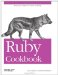# Representing Numbers to Arbitrary Precision

Problem

You're doing high- precision arithmetic, and floating-point numbers are not precise enough.

Solution

A BigDecimal number can represent a real number to an arbitrary number of decimal places.

```	require 'bigdecimal'

BigDecimal("10").to_s # => "0.1E2"
BigDecimal("1000").to_s # => "0.1E4"
BigDecimal("1000").to_s("F") # => "1000.0"

BigDecimal("0.123456789").to_s # => "0.123456789E0"
```

Compare how Float and BigDecimal store the same high-precision number:

```	nm = "0.123456789012345678901234567890123456789"
nm.to_f # => 0.123456789012346
BigDecimal(nm).to_s
# => "0.123456789012345678901234567890123456789E0"
```

Discussion

BigDecimal numbers store numbers in scientific notation format. A BigDecimal consists of a sign (positive or negative), an arbitrarily large decimal fraction, and an arbitrarily large exponent. This is similar to the way floating-point numbers are stored, but a double- precision floating-point implementation like Ruby's cannot represent an exponent less than Float::MIN_EXP (1021) or greater than Float::MAX_EXP (1024). Float objects also can't represent numbers at a greater precision than Float::EPSILON, or about 2.2*10-16.

You can use BigDecimal#split to split a BigDecimal object into the parts of its scientific-notation representation. It returns an array of four numbers: the sign (1 for positive numbers,1 for negative numbers), the fraction (as a string), the base of the exponent (which is always 10), and the exponent itself.

```	BigDecimal("105000").split
# => [1, "105", 10, 6]
# That is, 0.105*(10**6)

BigDecimal("-0.005").split
# => [-1, "5", 10, -2]
# That is, -1 * (0.5*(10**-2))
```

A good way to test different precision settings is to create an infinitely repeating decimal like 2/3, and see how much of it gets stored. By default, BigDecimals give 16 digits of precision, roughly comparable to what a Float can give.

```	(BigDecimal("2") / BigDecimal("3")).to_s
# => "0.6666666666666667E0"

2.0/3
# => 0.666666666666667
```

You can store additional significant digits by passing in a second argument n to the BigDecimal constructor. BigDecimal precision is allocated in chunks of four decimal digits. Values of n from 1 to 4 make a BigDecimal use the default precision of 16 digits. Values from 5 to 8 give 20 digits of precision, values from 9 to 12 give 24 digits, and so on:

```	def two_thirds(precision)
(BigDecimal("2", precision) / BigDecimal("3")).to_s
end

two_thirds(1) # => "0.6666666666666667E0"
two_thirds(4) # => "0.6666666666666667E0"
two_thirds(5) # => "0.66666666666666666667E0"
two_thirds(9) # => "0.666666666666666666666667E0"
two_thirds(13) # => "0.6666666666666666666666666667E0"
```

Not all of a number's significant digits may be used. For instance, Ruby considers BigDecimal("2") and BigDecimal("2.000000000000") to be equal, even though the second one has many more significant digits.

You can inspect the precision of a number with BigDecimal#precs. This method returns an array of two elements: the number of significant digits actually being used, and the toal number of significant digits. Again, since significant digits are allocated in blocks of four, both of these numbers will be multiples of four.

```	BigDecimal("2").precs # => [4, 8]
BigDecimal("2.000000000000").precs # => [4, 20]
BigDecimal("2.000000000001").precs # => [16, 20]
```

If you use the standard arithmetic operators on BigDecimals, the result is a BigDecimal accurate to the largest possible number of digits. Dividing or multiplying one BigDecimal by another yields a BigDecimal with more digits of precision than either of its parents, just as would happen on a pocket calculator.

```	(a = BigDecimal("2.01")).precs # => [8, 8]
(b = BigDecimal("3.01")).precs # => [8, 8]

(product = a * b).to_s("F") # => "6.0501"
product.precs # => [8, 24]
```

To specify the number of significant digits that should be retained in an arithmetic operation, you can use the methods add, sub, mul, and div instead of the arithmetic operators.

```	two_thirds = (BigDecimal("2", 13) / 3)
two_thirds.to_s # => "0.666666666666666666666666666666666667E0"

(two_thirds + 1).to_s # => "0.1666666666666666666666666666666666667E1"

```

Either way, BigDecimal math is significantly slower than floating-point math. Not only are BigDecimals allowed to have more significant digits than floats, but BigDecimals are stored as an array of decimal digits, while floats are stored in a binary encoding and manipulated with binary arithmetic.

The BigMath module in the Ruby standard library defines methods for performing arbitrary- precision mathematical operations on BigDecimal objects. It defines power-related methods like sqrt, log, and exp, and trigonometric methods like sin, cos, and atan.

All of these methods take as an argument a number prec indicating how many digits of precision to retain. They may return a BigDecimal with more than prec significant digits, but only prec of those digits are guaranteed to be accurate.

```	require 'bigdecimal/math'
include BigMath
two = BigDecimal("2")
BigMath::sqrt(two, 10).to_s("F") # => "1.4142135623730950488016883515"
```

That code gives 28 decimal places, but only 10 are guaranteed accurate (because we passed in an n of 10), and only 24 are actually accurate. The square root of 2 to 28 decimal places is actually 1.4142135623730950488016887242. We can get rid of the inaccurate digits with BigDecimal#round:

```	BigMath::sqrt(two, 10).round(10).to_s("F") # => "1.4142135624"
```

We can also get a more precise number by increasing n:

```	BigMath::sqrt(two, 28).round(28).to_s("F") # => "1.4142135623730950488016887242"
```

BigMath also annotates BigDecimal with class methods BigDecimal.PI and BigDecimal.E. These methods construct BigDecimals of those transcendental numbers at any level of precision.

```	Math::PI # => 3.14159265358979
Math::PI.class # => Float
BigDecimal.PI(1).to_s # => "0.31415926535897932364198143965603E1"
BigDecimal.PI(20).to_s
# => "0.3141592653589793238462643383279502883919859293521427E1"
```

• At the time of writing, BigMath::log was very slow for BigDecimals larger than about 10; see Recipe 2.7, "Taking Logarithms," for a much faster implementation
• See Recipe 2.4, "Representing Rational Numbers," if you need to exactly represent a rational number with an infinite decimal expansion, like 2/3
• The BigDecimal library reference is extremely useful; if you look at the generated RDoc for the Ruby standard library, BigDecimal looks almost undocumented, but it actually has a comprehensive reference file (in English and Japanese): it's just not in RDoc format, so it doesn't get picked up; this document is available in the Ruby source package, or do a web search for "BigDecimal: An extension library for Ruby"Ruby Cookbook (Cookbooks (OReilly))
ISBN: 0596523696
EAN: 2147483647
Year: N/A
Pages: 399